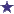# How many milligrams of sodium chloride are in a 250ml bag of 0.9% NaCl?

by Cheyenne
(Kissimmee, Florida)Sodium Chloride IV Fluids (250ml bag)

How many mg of sodium chloride are contained in a 250ml bag of 0.9% NaCl?

### Comments for How many milligrams of sodium chloride are in a 250ml bag of 0.9% NaCl?

Average RatingRatingHow many milligrams (mg) of Na+ in Normal Saline by: strum As an MD, far more pertinent than "how many milligrams of NaCl" would be how many mg (milligrams) of sodium per liter of 0.9% NaCl?There are 9 grams of NaCl in a liter bag of normal saline (NS), since NS is defined as 0.9% NS. The molecular weight of Na+ is ≈ 23 and that of Cl is 35. Therefore sodium is 40% of the NaCl. That would mean in a bag of NS containing 9 grams of NaCl, 40% would be sodium or 3.6 grams = 3,600 mg. Patients on a Na+ restricted diet are therefore getting 3,600 mg of Na+ per liter or 360 mg Na+ per 100 ml of normal saline.

 RatingIV Compounding Question 0.9% NaCl and Sodium Chloride by: David Great Question, Cheyenne! Each 100 milliliters of 0.9% NaCl contains 900 milligrams of Sodium Chloride, USP. For the 250ml bag of 0.9% NaCl, the amount of Sodium Chloride is 2.25 grams, or 2250 milligrams.

## Can someone help?

by Cheyenne
(Kissimmee, Florida)

how many mg of sodium chloride are contained in a 250ml bag of 0.9% NaCl?

### Comments for Can someone help?

Average RatingRatingSodium chloride by: Anonymous 9 mg sodium chloride per mL9 x 250 = 2250 mg